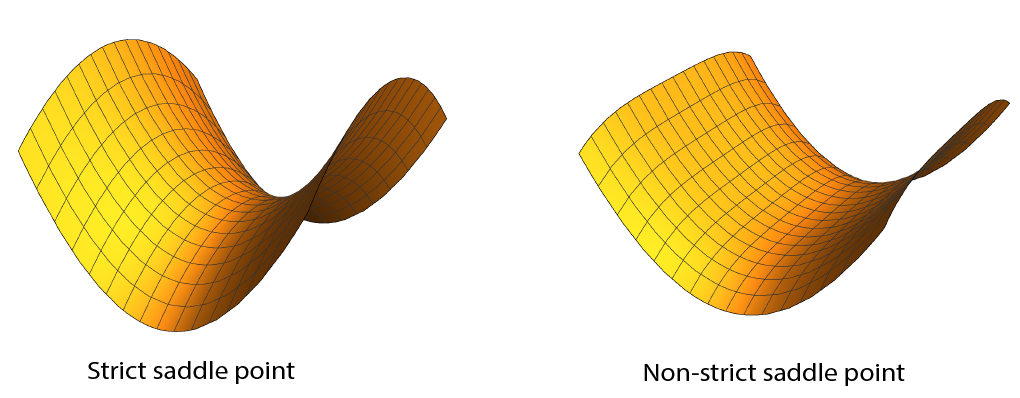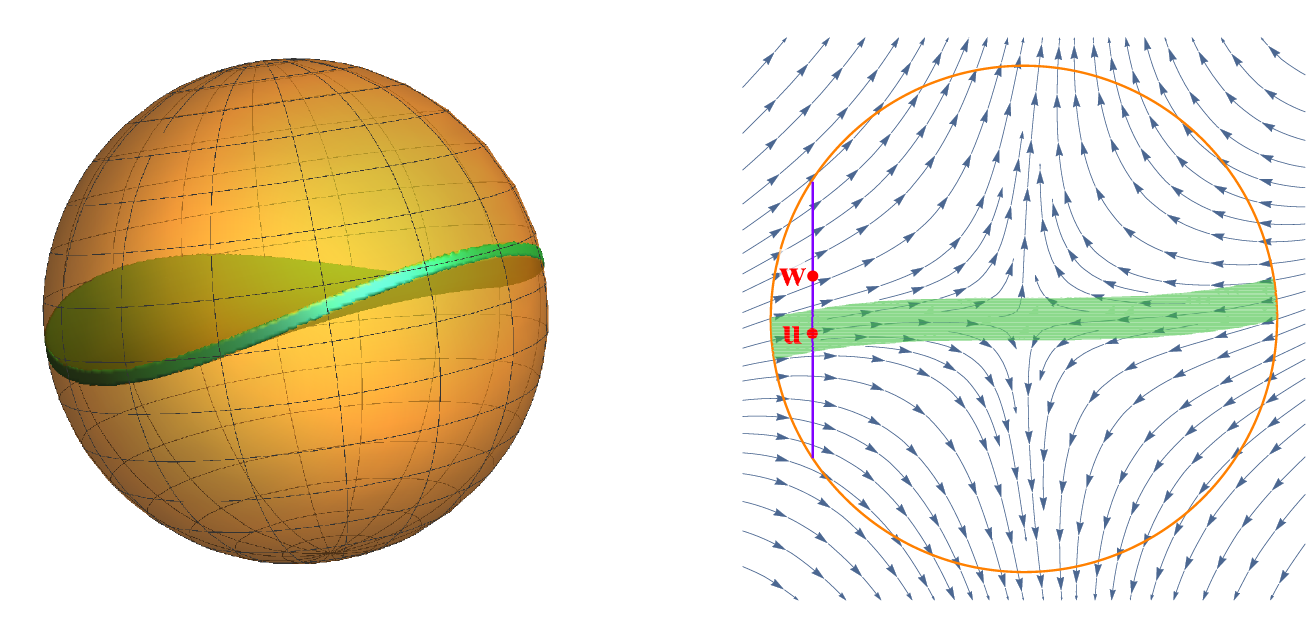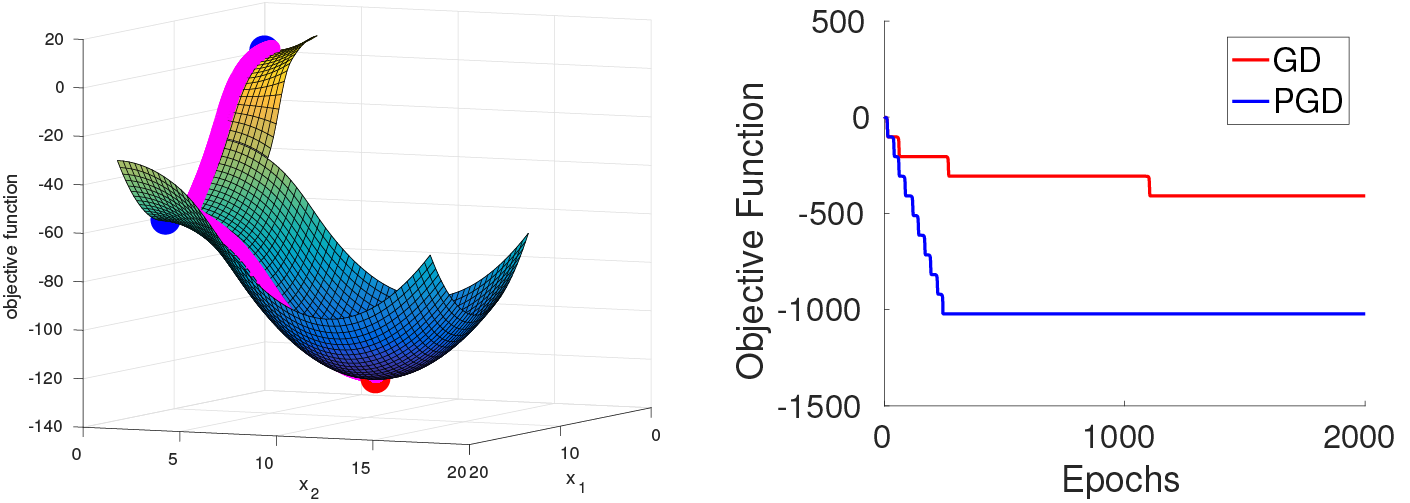This post was initially published on Off the Convex Path. It is reposted here with authors’ permission.

A core, emerging problem in nonconvex optimization involves the escape of saddle points. While recent research has shown that gradient descent (GD) generically escapes saddle points asymptotically (see Rong Ge’s and Ben Recht’s blog posts), the critical open problem is one of efficiency — is GD able to move past saddle points quickly, or can it be slowed down significantly? How does the rate of escape scale with the ambient dimensionality? In this post, we describe our recent work with Rong Ge, Praneeth Netrapalli and Sham Kakade, that provides the first provable positive answer to the efficiency question, showing that, rather surprisingly, GD augmented with suitable perturbations escapes saddle points efficiently; indeed, in terms of rate and dimension dependence it is almost as if the saddle points aren’t there!

We are in the realm of classical gradient descent (GD) — given a function $f:\mathbb{R}^d \to \mathbb{R}$ we aim to minimize the function by moving in the direction of the negative gradient:

$x_{t+1} = x_t - \eta \nabla f(x_t),$

where $x_t$ are the iterates and $\eta$ is the step size. GD is well understood theorietically in the case of convex optimization, but the general case of nonconvex optimization has been far less studied. We know that GD converges quickly to the neighborhood of stationary points (points where $\nabla f(x) = 0$) in the nonconvex setting, but these stationary points may be local minima or, unhelpfully, local maxima or saddle points.

Clearly GD will never move away from a stationary point if started there (even a local maximum); thus, to provide general guarantees, it is necessary to modify GD slightly to incorporate some degree of randomness. Two simple methods have been studied in the literature:

1. Intermittent Perturbations: Ge, Huang, Jin and Yuan 2015 considered adding occasional random perturbations to GD, and were able to provide the first polynomial time guarantee for GD to escape saddle points. (See also Rong Ge’s post )

2. Random Initialization: Lee et al. 2016 showed that with only random initialization, GD provably avoids saddle points asymptotically (i.e., as the number of steps goes to infinity). (see also Ben Recht’s post)

Asymptotic — and even polynomial time —results are important for the general theory, but they stop short of explaining the success of gradient-based algorithms in practical nonconvex problems. And they fail to provide reassurance that runs of GD can be trusted — that we won’t find ourselves in a situation in which the learning curve flattens out for an indefinite amount of time, with the user having no way of knowing that the asymptotics have not yet kicked in. Lastly, they fail to provide reassurance that GD has the kind of favorable properties in high dimensions that it is known to have for convex problems.

One reasonable approach to this issue is to consider second-order (Hessian-based) algorithms. Although these algorithms are generally (far) more expensive per iteration than GD, and can be more complicated to implement, they do provide the kind of geometric information around saddle points that allows for efficient escape. Accordingly, a reasonable understanding of Hessian-based algorithms has emerged in the literature, and positive efficiency results have been obtained.

Is GD also efficient? Or is the Hessian necessary for fast escape of saddle points?

A negative result emerges to this first question if one considers the random initialization strategy discussed. Indeed, this approach is provably inefficient in general, taking exponential time to escape saddle points in the worst case (see “On the Necessity of Adding Perturbations” section).

Somewhat surprisingly, it turns out that we obtain a rather different — and positive — result if we consider the perturbation strategy. To be able to state this result, let us be clear on the algorithm that we analyze:

1. for $~t = 1, 2, \ldots ~$ do
2. $\quad\quad x_{t} \leftarrow x_{t-1} - \eta \nabla f (x_{t-1})$
3. $\quad\quad$ if $~$perturbation condition holds$~$ then
4. $\quad\quad\quad\quad x_t \leftarrow x_t + \xi_t$

Here the perturbation $\xi_t$ is sampled uniformly from a ball centered at zero with a suitably small radius, and is added to the iterate when the gradient is suitably small. These particular choices are made for analytic convenience; we do not believe that uniform noise is necessary. nor do we believe it essential that noise be added only when the gradient is small.

## Strict-Saddle and Second-order Stationary Points

We define saddle points in this post to include both classical saddle points as well as local maxima. They are stationary points which are locally maximized along at least one direction. Saddle points and local minima can be categorized according to the minimum eigenvalue of Hessian:

$\lambda_{\min}(\nabla^2 f(x)) \begin{cases} > 0 \quad\quad \text{local minimum} \\ = 0 \quad\quad \text{local minimum or saddle point} \\ < 0 \quad\quad \text{saddle point} \end{cases}$

We further call the saddle points in the last category, where $\lambda_{\min}(\nabla^2 f(x)) < 0$, strict saddle points.While non-strict saddle points can be flat in the valley, strict saddle points require that there is at least one direction along which the curvature is strictly negative. The presence of such a direction gives a gradient-based algorithm the possibility of escaping the saddle point. In general, distinguishing local minima and non-strict saddle points is NP-hard; therefore, we — and previous authors — focus on escaping strict saddle points.

Formally, we make the following two standard assumptions regarding smoothness.

Assumption 1: $f$ is $\ell$-gradient-Lipschitz, i.e.
$\quad\quad\quad\quad \forall x_1, x_2, |\nabla f(x_1) - \nabla f(x_2)| \le \ell |x_1 - x_2|$.
$~$
Assumption 2: $f$ is $\rho$-Hessian-Lipschitz, i.e.
$\quad\quad\quad\quad \forall x_1, x_2$, $|\nabla^2 f(x_1) - \nabla^2 f(x_2)| \le \rho |x_1 - x_2|$.

Similarly to classical theory, which studies convergence to a first-order stationary point, $\nabla f(x) = 0$, by bounding the number of iterations to find a $\epsilon$-first-order stationary point, $|\nabla f(x)| \le \epsilon$, we formulate the speed of escape of strict saddle points and the ensuing convergence to a second-order stationary point, $\nabla f(x) = 0, \lambda_{\min}(\nabla^2 f(x)) \ge 0$, with an $\epsilon$-version of the definition:

Definition: A point $x$ is an $\epsilon$-second-order stationary point if:
$\quad\quad\quad\quad |\nabla f(x)|\le \epsilon$, and $\lambda_{\min}(\nabla^2 f(x)) \ge -\sqrt{\rho \epsilon}$.

In this definition, $\rho$ is the Hessian Lipschitz constant introduced above. This scaling follows the convention of Nesterov and Polyak 2006.

### Applications

In a wide range of practical nonconvex problems it has been proved that all saddle points are strict — such problems include, but not are limited to, principal components analysis, canonical correlation analysis, orthogonal tensor decomposition, phase retrieval, dictionary learning, matrix sensing, matrix completion, and other nonconvex low-rank problems.

Furthermore, in all of these nonconvex problems, it also turns out that all local minima are global minima. Thus, in these cases, any general efficient algorithm for finding $\epsilon$-second-order stationary points immediately becomes an efficient algorithm for solving those nonconvex problem with global guarantees.

In the classical case of first-order stationary points, GD is known to have very favorable theoretical properties:

Theorem (Nesterov 1998): If Assumption 1 holds, then GD, with $\eta = 1/\ell$, finds an $\epsilon$-first-order stationary point in $2\ell (f(x_0) - f^\star)/\epsilon^2$ iterations.

In this theorem, $x_0$ is the initial point and $f^\star$ is the function value of the global minimum. The theorem says for that any gradient-Lipschitz function, a stationary point can be found by GD in $O(1/\epsilon^2)$ steps, with no explicit dependence on $d$. This is called “dimension-free optimization” in the literature; of course the cost of a gradient computation is $O(d)$, and thus the overall runtime of GD scales as $O(d)$. The linear scaling in $d$ is especially important for modern high-dimensional nonconvex problems such as deep learning.

We now wish to address the corresponding problem for second-order stationary points. What is the best we can hope for? Can we also achieve

1. A dimension-free number of iterations;
2. An $O(1/\epsilon^2)$ convergence rate;
3. The same dependence on $\ell$ and $(f(x_0) - f^\star)$ as in (Nesterov 1998)?

Rather surprisingly, the answer is Yes to all three questions (up to small log factors).

Main Theorem: If Assumptions 1 and 2 hold, then PGD, with $\eta = O(1/\ell)$, finds an $\epsilon$-second-order stationary point in $\tilde{O}(\ell (f(x_0) - f^\star)/\epsilon^2)$ iterations with high probability.

Here $\tilde{O}(\cdot)$ hides only logarithmic factors; indeed, the dimension dependence in our result is only $\log^4(d)$. The theorem thus asserts that a perturbed form of GD, under an additional Hessian-Lipschitz condition, converges to a second-order-stationary point in almost the same time required for GD to converge to a first-order-stationary point. In this sense, we claim that PGD can escape strict saddle points almost for free.

We turn to a discussion of some of the intuitions underlying these results.

### Why do polylog(d) iterations suffice?

Our strict-saddle assumption means that there is only, in the worst case, one direction in $d$ dimensions along which we can escape. A naive search for the descent direction intuitively should take at least $\text{poly}(d)$ iterations, so why should only $\text{polylog}(d)$ suffice?

Consider a simple case in which we assume that the function is quadratic in the neighborhood of the saddle point. That is, let the objective function be $f(x) = x^\top H x$, a saddle point at zero, with constant Hessian $H = \text{diag}(-1, 1, \cdots, 1)$. In this case, only the first direction is an escape direction (with negative eigenvalue $-1$).

It is straightforward to work out the general form of the iterates in this case:

$x_t = x_{t-1} - \eta \nabla f(x_{t-1}) = (I - \eta H)x_{t-1} = (I - \eta H)^t x_0.$

Assume that we start at the saddle point at zero, then add a perturbation so that $x_0$ is sampled uniformly from a ball $\mathcal{B}_0(1)$ centered at zero with radius one. The decrease in the function value can be expressed as:

$f(x_t) - f(0) = x_t^\top H x_t = x_0^\top (I - \eta H)^t H (I - \eta H)^t x_0.$

Set the step size to be $1/2$, let $\lambda_i$ denote the $i$-th eigenvalue of the Hessian $H$ and let $\alpha_i = e_i^\top x_0$ denote the component in the $i$th direction of the initial point $x_0$. We have $\sum_{i=1}^d \alpha_i^2 = | x_0|^2 = 1$, thus:

$f(x_t) - f(0) = \sum_{i=1}^d \lambda_i (1-\eta\lambda_i)^{2t} \alpha_i^2 \le -1.5^{2t} \alpha_1^2 + 0.5^{2t}.$

A simple probability argument shows that sampling uniformly in $\mathcal{B}_0(1)$ will result in at least a $\Omega(1/d)$ component in the first direction with high probability. That is, $\alpha^2_1 = \Omega(1/d)$. Substituting $\alpha_1$ in the above equation, we see that it takes at most $O(\log d)$ steps for the function value to decrease by a constant amount.

### Pancake-shape stuck region for general Hessian

We can conclude that for the case of a constant Hessian, only when the perturbation $x_0$ lands in the set $\{x | ~ |e_1^\top x|^2 \le O(1/d)\}$ $\cap \mathcal{B}_0 (1)$, can we take a very long time to escape the saddle point. We call this set the stuck region; in this case it is a flat disk. In general, when the Hessian is no longer constant, the stuck region becomes a non-flat pancake, depicted as a green object in the left graph. In general this region will not have an analytic expression.

Earlier attempts to analyze the dynamics around saddle points tried to the approximate stuck region by a flat set. This results in a requirement of an extremely small step size and a correspondingly very large runtime complexity. Our sharp rate depends on a key observation — although we don’t know the shape of the stuck region, we know it is very thin.In order to characterize the “thinness” of this pancake, we studied pairs of hypothetical perturbation points $w, u$ separated by $O(1/\sqrt{d})$ along an escaping direction. We claim that if we run GD starting at $w$ and $u$, at least one of the resulting trajectories will escape the saddle point very quickly. This implies that the thickness of the stuck region can be at most $O(1/\sqrt{d})$, so a random perturbation has very little chance to land in the stuck region.

## On the Necessity of Adding Perturbations

We have discussed two possible ways to modify the standard gradient descent algorithm, the first by adding intermittent perturbations, and the second by relying on random initialization. Although the latter exhibits asymptotic convergence, it does not yield efficient convergence in general; in recent joint work with Simon Du, Jason Lee, Barnabas Poczos, and Aarti Singh, we have shown that even with fairly natural random initialization schemes and non-pathological functions, GD with only random initialization can be significantly slowed by saddle points, taking exponential time to escape. The behavior of PGD is strikingingly different — it can generically escape saddle points in polynomial time.

To establish this result, we considered random initializations from a very general class including Gaussians and uniform distributions over the hypercube, and we constructed a smooth objective function that satisfies both Assumptions 1 and 2. This function is constructed such that, even with random initialization, with high probability both GD and PGD have to travel sequentially in the vicinity of $d$ strict saddle points before reaching a local minimum. All strict saddle points have only one direction of escape. (See the left graph for the case of $d=2$).When GD travels in the vicinity of a sequence of saddle points, it can get closer and closer to the later saddle points, and thereby take longer and longer to escape. Indeed, the time to escape the $i$th saddle point scales as $e^{i}$. On the other hand, PGD is always able to escape any saddle point in a small number of steps independent of the history. This phenomenon is confirmed by our experiments; see, for example, an experiment with $d=10$ in the right graph.

## Conclusion

In this post, we have shown that a perturbed form of gradient descent can converge to a second-order-stationary point at almost the same rate as standard gradient descent converges to a first-order-stationary point. This implies that Hessian information is not necessary for to escape saddle points efficiently, and helps to explain why basic gradient-based algorithms such as GD (and SGD) work surprisingly well in the nonconvex setting. This new line of sharp convergence results can be directly applied to nonconvex problem such as matrix sensing/completion to establish efficient global convergence rates.

There are of course still many open problems in general nonconvex optimization. To name a few: will adding momentum improve the convergence rate to a second-order stationary point? What type of local minima are tractable and are there useful structural assumptions that we can impose on local minima so as to avoid local minima efficiently? We are making slow but steady progress on nonconvex optimization, and there is the hope that at some point we will transition from “black art” to “science”.